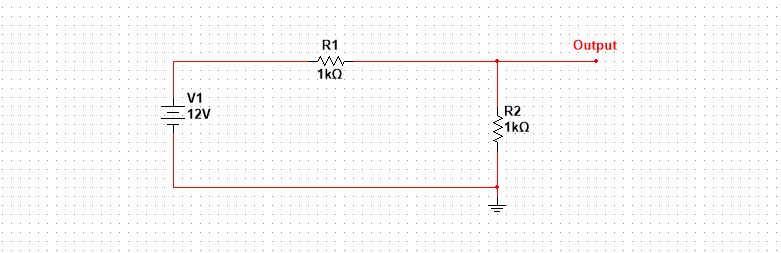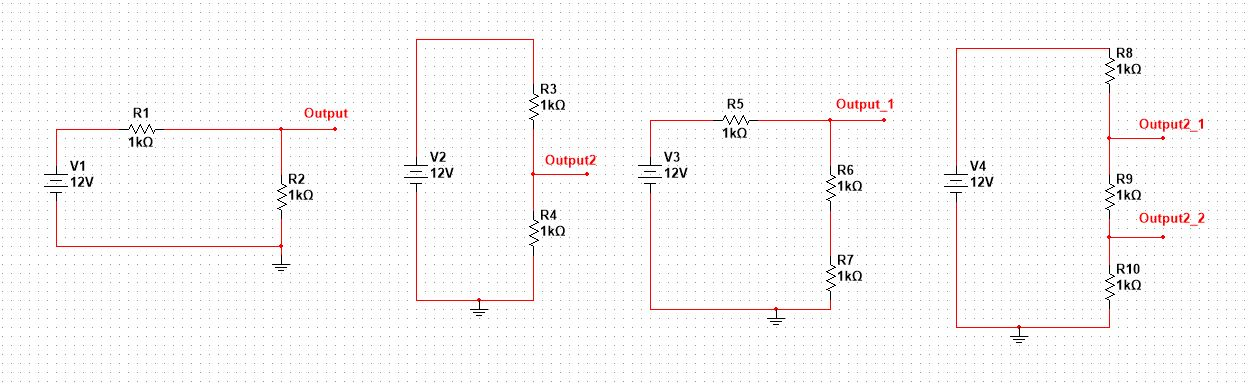# How to design a resistor voltage divider [Step by step]

Hey there, looking for a voltage divider design? The practical design, not the theory. Then welcome, here in this post I going to share how to design a voltage divider.

## Voltage divider design

A voltage divider is a very basic circuit when it comes to circuit design. It plays a key role when different levels of voltages are required with in the same circuit. As the name suggests, voltage divider, the circuit that divides the input voltage into the required levels.

A voltage divider is a general term, one can use a potentiometer for voltage division, but the type we will restrict ourselves in this post is resistors based voltage division.

Let’s have a look at this guy.Look at this guy, it is so damn ugly and confusing. I know, but this is the most simple one in the entire circuit design. And by the way, I used Multisim simulation software for this circuit.

An above circuit is just a simple form of a voltage divider. Let’s see how this guy can confuse us in different forms.The output voltage can be between a node and reference ground, or it may be the difference between two nodes as well (see the last diagram). No matter how many resistors are being used, remember if they at the end making a series type connection, chances are it might be a voltage divider.

There are so many ways you can use it according to your application, but most generally it is used as a reference voltage. In simple, it is best used to make a reference voltage level.

The accuracy of the output voltage is amazing if we play nice when selecting load, or resistor values. Selecting load is something we mostly can’t control, but we are in control of designing the divider resistance values.

Alright!

Let’s jump into the actual design.

But before let’s derive the design equation first. Excuse my bad handwriting below.The above formula is the backbone of the design procedure. Try to derive it once yourselves and try to understand it. Hopefully, you will have fun. I know, some of you would say, Yaman it is not fun, it is that damn boring maths.

Alright!

### Step 1: Define the output voltage

The first step in voltage divider design is to define the output voltage. Like, for which voltage level you want to design the divider circuit.

Mostly, the divider circuit is used for voltage reference. Let say we want a reference voltage of 3.3V for our application, then 3.3V is our required output for the divider circuit.

Always keep in mind, voltage divider should not be used for powering up devices. It is not meant for powering high power devices. Sure you can use it for low power devices like LEDs.

The reason is, it’s not stable. High power devices need high current, which means high heat across R1. This may lead to burning the poor resistor.

So, now you have two known i.e. the input voltage and the output voltage. All we left is with the two unknown resistor values.

### Step 2: Calculating the resistor values

This is a fun task. In theory, you can design any voltage divider circuit, but in reality, you are limited.

You can’t choose random resistor values, because these values must be available in the market to practically implement the circuit, i.e. they must be standard values.

For example, you choose random values, go to the store and find out no such values exist. Congrats, you just designed a very poor divider circuit. Now, some of you would say, Yaman we can use series and parallel to get the value of our desire. My answer, true, very true. But it will make your design ugly and full of resistors and a lot of energy wastage.

You can’t use the low resistor values. The reason is, we don’t want any power loss at the divider circuit. We want it just as a constant voltage reference.

Now, the above two things are very important. Keep them in your mind.

Select the value of R2 first then calculate for the value of R1.

Choose the values in Kilo. Don’t go for low resistor values, it will cause power loss in the circuit.

I think it is time for some examples to make it a little more clear.

Example: Design a voltage divider circuit for the required output voltage of 5V, the input voltage is 10V.

First thing, note the input and output voltages, the output is half the input. So, keeping the voltage divider equation in mind, it is only possible if$R_2=R_1$.$Vout=Vin [R_2/R_2+R_2] \\ Vout=Vin [1/2] \\ Vout = 10/2 =5V$

Second, try to choose standard values within the range of kiloOhms. So, the best values for this case are 10kOhms.

There are many other values possible, you can go with that as well. But remember we have not connected this to the load yet, and load is another factor to be considered when choosing values for resistors.

Other useful posts

## Amazing Student Deal

Experience the World’s Largest Library of Audiobooks from Amazon.

• 30 days of membership free, plus 1 audiobook and 2 Audible Originals to get you started.
• After trial, you’ll get 3 titles each month: 1 audiobook and 2 Audible Originals of your choice.
• Quick & easy exchanges. Swap any audiobook you don’t love.
• Cancel online anytime, but keep your entire library forever.###### 3 Best basic electronics books [Latest & Affordable]

Hay there! I hope you are having a great time. Looking for some basic electronics books? Well then, welcome. Every book is great. If